# Free Fall

0
4429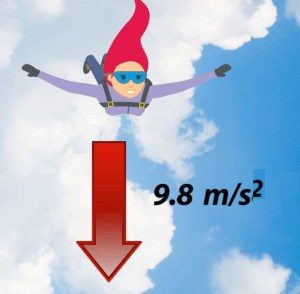If some body is being dropped, it will start falling down towards the Earth’s surface. It has been determined experimentally that all bodies have the same speed if they fall through the space that has no air. Any two bodies released at the same time from the same height in a vacuum space would fall to the Earth at the same time. If some body is being dropped, it will start falling down towards the Earth’s surface.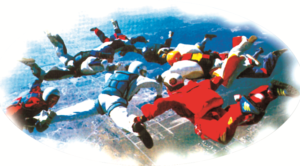It has been determined experimentally that all bodies have the same speed if they fall through the vacuum space. In vacuum space, any two bodies released at the same time from the same height would fell on the Earth’s surface at the same time. The described movement is called a free fall.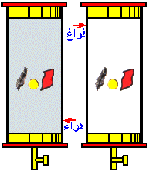Example: Free fall in vacuum can be demonstrated using the Newton’s tube. If there is an air inside the tube and we drop a metal ball and a feather at the same time, we will see that the metal coin will fall faster than the feather.

However, if the air is drawn from the tube in which the bodies are located, there will be no more air resistance. Only the force of gravity acts in the vacuum space, thus the bodies in free fall have the same acceleration “g”,which is why the ball and the feather would fall at the bottom of the pipe at the same speed.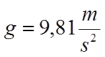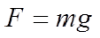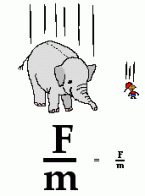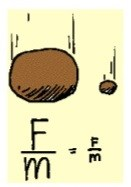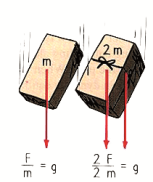The acceleration of the free fall is not the same at all places on the Earth, and it increasing from the equator to the pole. At 45 ° latitude near the Earth’s surface, the acceleration g = 9.81 m / s².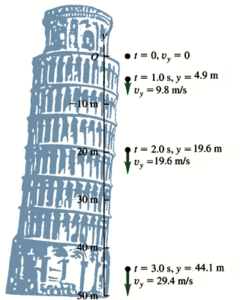free fall is a uniformly accelerated movement without a starting speed. The picture shows the distance of the traveled path and the speed after 1, 2 and 3 seconds of free fall. Th speed increases every second for the same value. Also, the length of the traveled path for each second is increased.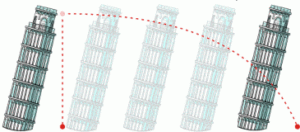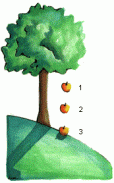The dependence among the sizes that characterize this movement is given by the equations for uniformly accelerated motion, where instead of “a” for acceleration, we denote it using  “g”

(gravitational acceleration), and the path traveled is denoted by “h” instead of “s”.

speed: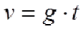or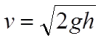traveled path: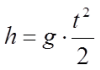or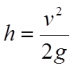Example: If we drop the stone to the bottom of the well, after 4 seconds we hear a blow.

Calculate the depth of the well.

t = 4 s

g = 9.81 m / s²

h =?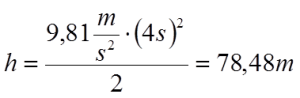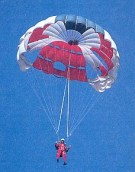When the body falls through some medium, then next to the Earth’s gravity, we must also take into account the force of the air’s resistance. Objects of different shapes and sizes fall at different speeds. Let’s take the parachute as an example. A body without a parachute would fall at a very at a high speed, but if a body has an open parachute attached, it would descend to the ground slowly because the body’s speed is relatively small due to air resistance even though the ground forces are more difficult.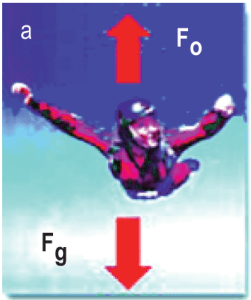Two forces act on the falling body: force Fg in downward direction and air resistance Fo in the opposite direction. The intensity of the resulting force is equal to the difference in intensity of gravity force and the air resistance.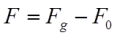In case of the free fall, the amount of force is steadily constant, while the amount of resistance of the air force increases with speed.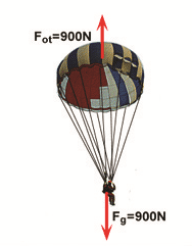Example: When moving of the parachute, the gravitational force and the resistance of the air force act as shown in the figure. What is the resultant force that affects the parachute?

Fv = 900 N

G = 900 N

————-

Fr = 900 N – 900 N = 0; Acceleration is zero, because the resulting force is zero. Parachutist falls at a steady pace.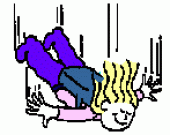An object in the technical sense of the term “free fall” may not necessarily be falling down in the usual sense of the term. An object moving upwards would not normally be considered to be falling, but if it is subject to the force of gravity only, it is said to be in free fall.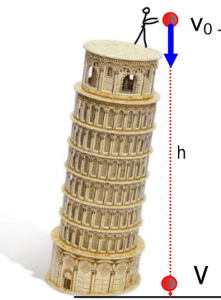Vertical Trajectory

If we throw a body with an initial velocity v0 downwards, it will move uniformly with the action of the force of gravity, and therefore the speed:

where v0 is the initial velocity that is given to the body when it is being thrown. If with “h” we indicate the height that the body has reached at some point “t”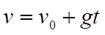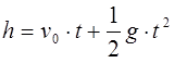Trajectory Upwards is the uniformly slow motion of a body that is thrown vertically upward from a certain height or from the surface of the Earth with an initial speed.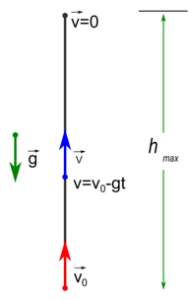It is a movement with two stages – in the first stage, the body has uniformly accelerated movement, and in the second stage the body falls freely.

The speed of the body v in case of the upward trajetory changes, as it moves up, in the following way:

where v0 is the initial velocity of the body as it is thrown. If the “h” indicates the height that the body has reached at some point “t”

For downward direction:

For upward direction:

We need to denote the up and down direction

We are going to use + for up and – for down. The + and – are just to identify direction, they have nothing to do with the magnitude.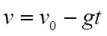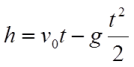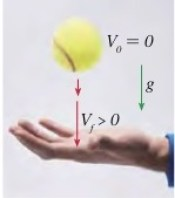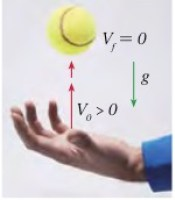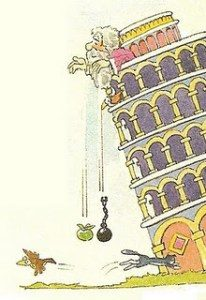Galileo Galileo was examining the free fall of the body on top of the leaning tower in Pisa. He dropped various objects from the tower and concluded that the bodies were getting different acceleration due to the air resistance. He concluded that the bodies in the vacuum space would fall at the same speed, which was later on confirmed experimentally.

For instance, Aristotle claimed that the bodies fell on the ground at a rate proportional to their mass, but Galileo proved that it was not true. Actually, some say that that Galileo threw the bodies from the top of the famous leaning tower in Pisa in order to prove that Aristotle was wrong, but this story is generally considered to be false.

Galileo performed and experiment where he was measuring the speed of the bodies after measuring their mass. He dropped two bodies of the same dimensions and different masses down the steep slopes and the results showed against Aristotle’s claims.

SHARE
Previous articleNewton’s Law of Gravity